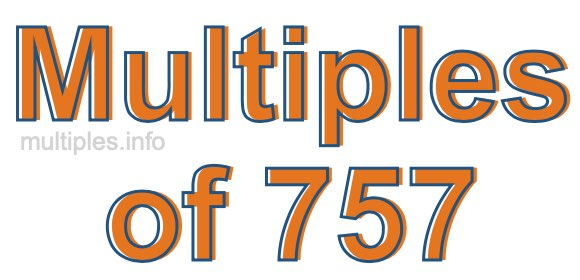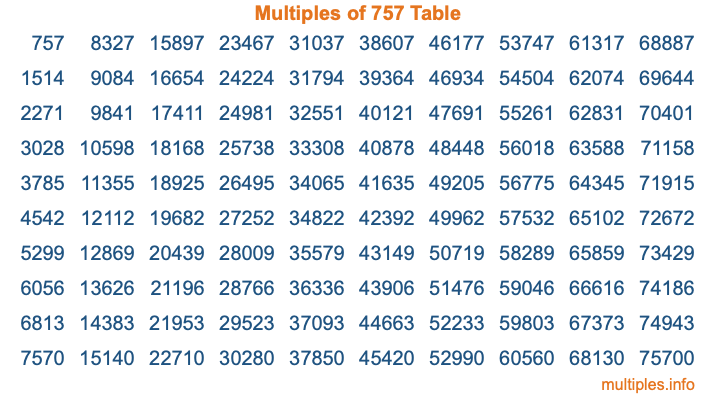Multiples of 757Welcome to the Multiples of 757 page. Here we will first teach you everything you will ever need to know about the multiples of 757, and then give you a study guide summary of everything we taught you to make sure you remember it all. Use this page to look up facts and learn information about the multiples of 757. This page will make you a multiples of seven hundred fifty-seven expert!

Definition of Multiples of 757
Multiples of 757 are all the numbers that when divided by 757 equal an integer. Each of the multiples of 757 are called a multiple. A multiple of 757 is created by multiplying 757 by an integer.

Therefore, to create a list of multiples of 757, you start with 1 multiplied by 757, then 2 multiplied by 757, then 3 multiplied by 757, and so on for as long as you want. Thus, the list of the first five multiples of 757 is 757, 1514, 2271, 3028, and 3785. To see a larger list of multiples of 757, see the printable image of Multiples of 757 further down on this page. We also have a category where you can choose any nth multiple of 757.

Multiples of 757 Checker
The Multiples of 757 Checker below checks to see if any number of your choice is a multiple of 757. In other words, it checks to see if there is any number (integer) that when multiplied by 757 will equal your number. To do that, we divide your number by 757. If the the quotient is an integer, then your number is a multiple of 757.

Is  a multiple of 757?

Least Common Multiple of 757 and ...
A Least Common Multiple (LCM) is the lowest multiple that two or more numbers have in common. This is also called the smallest common multiple or lowest common multiple and is useful to know when you are adding our subtracting fractions. Enter one or more numbers below (757 is already entered) to find the LCM.

Check out our LCM Calculator if you need more details about the Least Common Multiple or if you need the LCM for different numbers for adding and subtraction fractions.

nth Multiple of 757
As we stated above, 757 is the first multiple of 757, 1514 is the second multiple of 757, 2271 is the third multiple of 757, and so on. Enter a number below to find the nth multiple of 757.

th multiple of 757

Multiples of 757 vs Factors of 757
757 is a multiple of 757 and a factor of 757, but that is where the similarities end. All postive multiples of 757 are 757 or greater than 757. All positive factors of 757 are 757 or less than 757.

Below is the beginning list of multiples of 757 and the factors of 757 so you can compare:

Multiples of 757: 757, 1514, 2271, 3028, 3785, etc.

Factors of 757: 1, 757

As you can see, the multiples of 757 are all the numbers that you can divide by 757 to get a whole number. The factors of 757, on the other hand, are all the whole numbers that you can multiply by another whole number to get 757.

It's also interesting to note that if a number (x) is a factor of 757, then 757 will also be a multiple of that number (x).

Multiples of 757 vs Divisors of 757
The divisors of 757 are all the integers that 757 can be divided by evenly. Below is a list of the divisors of 757.

Divisors of 757: 1, 757

The interesting thing to note here is that if you take any multiple of 757 and divide it by a divisor of 757, you will see that the quotient is an integer.

Multiples of 757 Table
Below is an image of the first 100 multiples of 757 in a table. The table is in chronological order, column by column. The first column has the first ten multiples of 757, the second column has the next ten multiples of 757, and so on.The Multiples of 757 Table is also referred to as the 757 Times Table or Times Table of 757. You are welcome to print out our table for your studies.

Negative Multiples of 757
Although not often discussed or needed in math, it is worth mentioning that you can make a list of negative multiples of 757 by multiplying 757 by -1, then by -2, then by -3, and so on, to get the following list of negative multiples of 757:

-757, -1514, -2271, -3028, -3785, etc.

Multiples of 757 Summary
Below is a summary of important Multiples of 757 facts that we have discussed on this page. To retain the knowledge on this page, we recommend that you read through the summary and explain to yourself or a study partner why they hold true.

There are an infinite number of multiples of 757.

A multiple of 757 divided by 757 will equal a whole number.

757 divided by a factor of 757 equals a divisor of 757.

The nth multiple of 757 is n times 757.

The largest factor of 757 is equal to the first positive multiple of 757.

757 is a multiple of every factor of 757.

757 is a multiple of 757.

A multiple of 757 divided by a divisor of 757 equals an integer.

757 divided by a divisor of 757 equals a factor of 757.

Any integer times 757 will equal a multiple of 757.

Multiples of a Number
Here you can get the multiples of another number, all with the same attention to detail as we did for multiples of 757 on this page.

Multiples of
Multiples of 758
Did you find our page about multiples of seven hundred fifty-seven educational? Do you want more knowledge? Check out the multiples of the next number on our list!